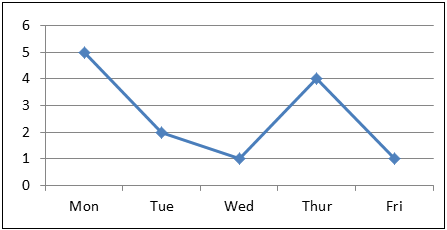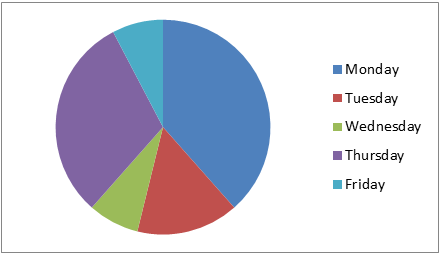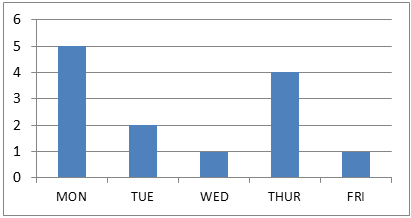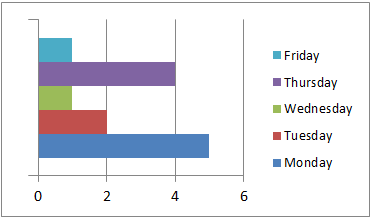QuestionAnswers

# _____________ represents data that changes continuously over a period of time.${\text{A)}}$ Line Graph${\text{B)}}$ Pie Graph ${\text{C)}}$ Bar Graph${\text{D)}}$ HistogramVerified
92.7k+ views
Hint: Here we discuss the given option one by one and then we conclude the required answer. Also, we discuss each of the examples in the given option; it helps to get the required answer.

Complete step-by-step solution:
Line graph: When the given quantitative data is in individual series, we use line graphs. Line graphs are probably used to represent the individual series with a single line consisting of dots. The line is drawn by connecting the dots. It helps us represent data that changes frequently over the period of time. Line graphs are also known as line chart
Example:
 Monday Tuesday Wednesday Thursday Friday $5$ $2$ $1$ $4$ $1$In this graph, it clearly depicts the changes occurring each day.
Pie graph: Pie graph is a very commonly used type of graph to represent things in circular form which is actually divided into different pieces or slices to represent the numerical values of the data. In the pie graph, the pieces length is known as arc length. The data given will be converted into degrees and represented in the table.
Example
The given data is:
 Monday Tuesday Wednesday Thursday Friday $5$ $2$ $1$ $4$ $1$Bar graph: Bar graph is the one of the most frequently used graphs to represent statistical data or numerical values. Bar graphs are usually represented by rectangular blocks or bars to categorise each set of data. They are plotted both vertically and horizontally according to the presenter’s will.
Example
Let this be the given data:
 Monday Tuesday Wednesday Thursday Friday $5$ $2$ $1$ $4$ $1$Histogram: Histogram can be also called or said as a family of bar graphs. It is because histogram contains many features of the bar graph. It is often used to show the height of each block or bars to compare. Simply it is a graphical representation of data using the bars that are in different heights.
Example:
 Monday Tuesday Wednesday Thursday Friday $5$ $2$ $1$ $4$ $1$So, the correct answer is line graph.

Option A is the correct answer.

Note: Change in data continuously does not mean the difference in changes of each value among them or the differences between each value how one or more variables vary or changes over a continuous period of time. Students may go wrong in understanding the sentences given in the question and get confused.